# Estimate Numbers: Where is the Arrow Pointing?

In this worksheet, students estimate the number the arrow points to.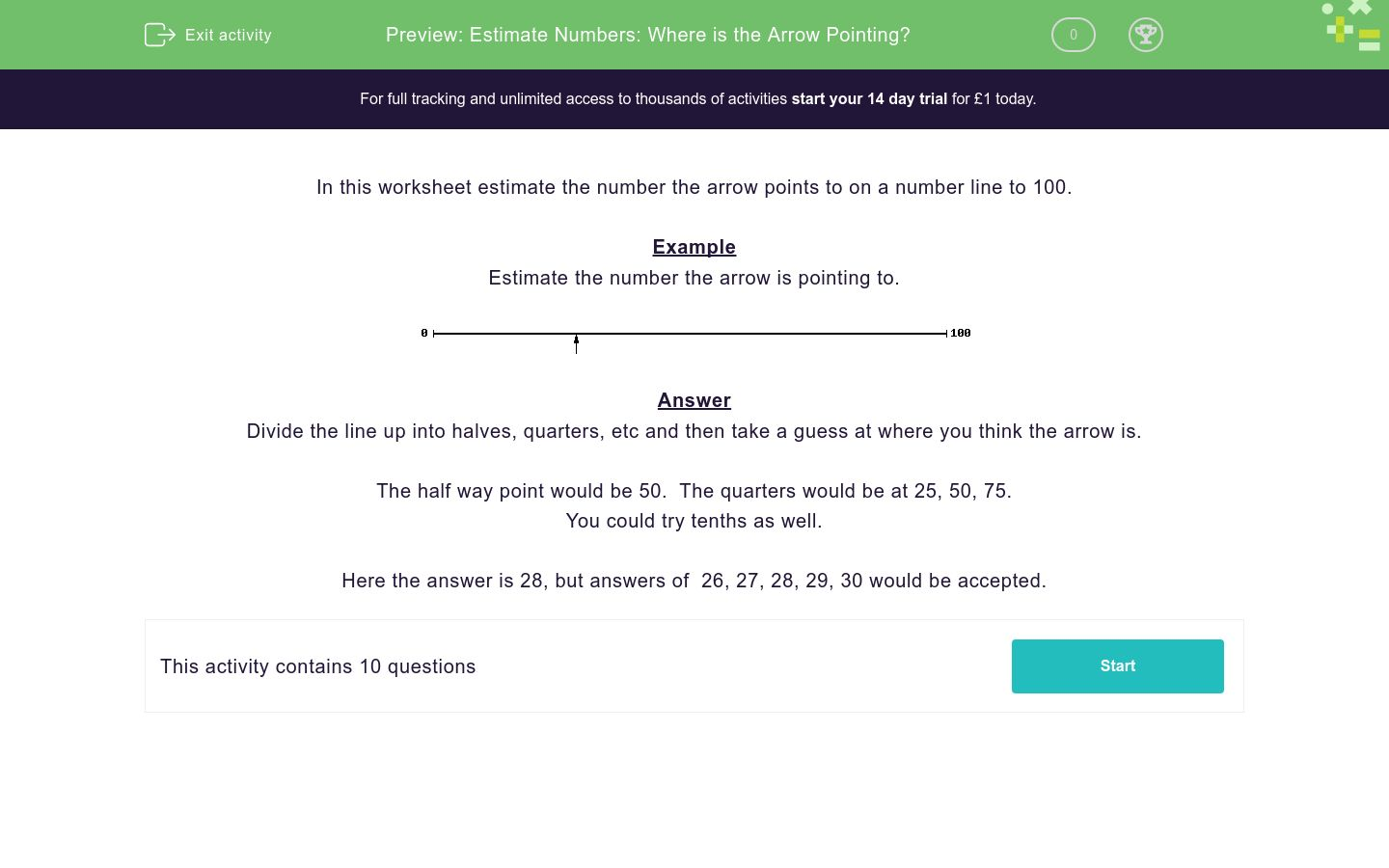Key stage:  KS 2

Curriculum topic:   Number: Number and Place Value

Curriculum subtopic:   Represent Numbers to 1000

Difficulty level:### QUESTION 1 of 10

In this worksheet estimate the number the arrow points to on a number line to 100.

Example

Estimate the number the arrow is pointing to.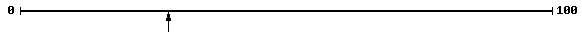Divide the line up into halves, quarters, etc and then take a guess at where you think the arrow is.

The half way point would be 50.  The quarters would be at 25, 50, 75.

You could try tenths as well.

Here the answer is 28, but answers of  26, 27, 28, 29, 30 would be accepted.

Estimate the number the arrow is pointing to.Estimate the number the arrow is pointing to.Estimate the number the arrow is pointing to.Estimate the number the arrow is pointing to.Estimate the number the arrow is pointing to.Estimate the number the arrow is pointing to.Estimate the number the arrow is pointing to.Estimate the number the arrow is pointing to.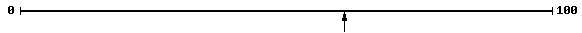Estimate the number the arrow is pointing to.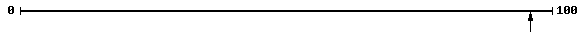Estimate the number the arrow is pointing to.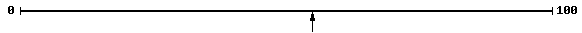• Question 1

Estimate the number the arrow is pointing to.47
48
49
50
51
EDDIE SAYS
The arrow is almost in the middle of the number line, so acceptable answers are: 47, 48, 49, 50 and 51.
• Question 2

Estimate the number the arrow is pointing to.40
41
42
43
44
EDDIE SAYS
The arrow is a bit to the left of the center of the number line, so acceptable answers are: 40, 41, 42, 43 and 44.
• Question 3

Estimate the number the arrow is pointing to.34
35
36
37
38
EDDIE SAYS
If the number line was divided into tens, the arrow is pointing in the thirties, so acceptable answers are: 34, 35, 36, 37 and 38.
• Question 4

Estimate the number the arrow is pointing to.1
2
3
4
5
EDDIE SAYS
The arrow is almost at the beginning of the number line, so acceptable answers are: 1, 2, 3, 4 and 5.
• Question 5

Estimate the number the arrow is pointing to.32
33
34
35
36
EDDIE SAYS
If the number line was divided into tens, the arrow is pointing in the thirties, so acceptable answers are: 32, 33, 34, 35 and 36.
• Question 6

Estimate the number the arrow is pointing to.50
51
52
53
54
EDDIE SAYS
The arrow is in the middle of the number line, so acceptable answers are: 50, 51, 52, 53 and 54.
• Question 7

Estimate the number the arrow is pointing to.33
34
35
36
37
EDDIE SAYS
If the number line was divided into tens, the arrow is pointing in the thirties, so acceptable answers are: 33, 34, 35, 36 and 37.
• Question 8

Estimate the number the arrow is pointing to.59
60
61
62
63
EDDIE SAYS
The arrow is pointing to the right of the middle of the number line, so acceptable answers are: 59, 60, 61, 62 and 63.
• Question 9

Estimate the number the arrow is pointing to.94
95
96
97
98
EDDIE SAYS
If the number line was divided into tens, the arrow is pointing in the nineties, so acceptable answers are: 94, 95, 96, 97 and 98.
• Question 10

Estimate the number the arrow is pointing to.53
54
55
56
57
EDDIE SAYS
The arrow is pointing slightly right of the middle, so acceptable answers are: 53, 54, 55, 56 and 57.
---- OR ----

Sign up for a £1 trial so you can track and measure your child's progress on this activity.

### What is EdPlace?

We're your National Curriculum aligned online education content provider helping each child succeed in English, maths and science from year 1 to GCSE. With an EdPlace account you’ll be able to track and measure progress, helping each child achieve their best. We build confidence and attainment by personalising each child’s learning at a level that suits them.

Get started